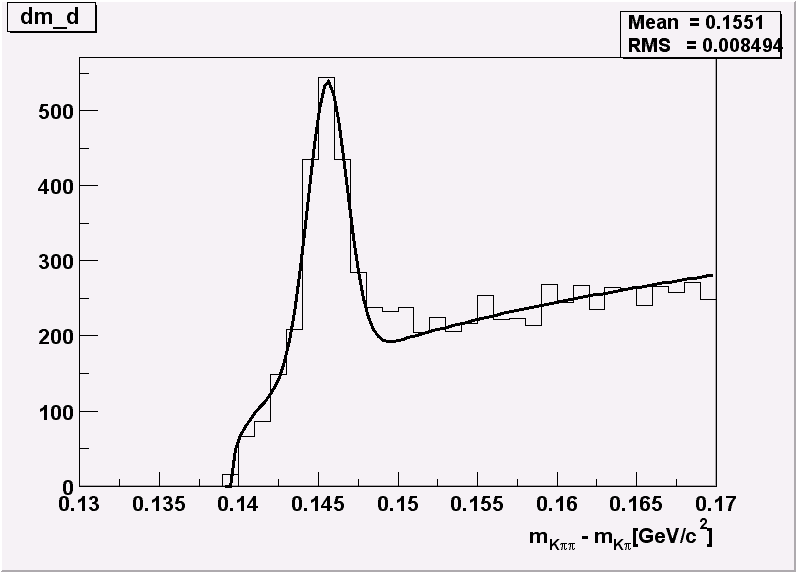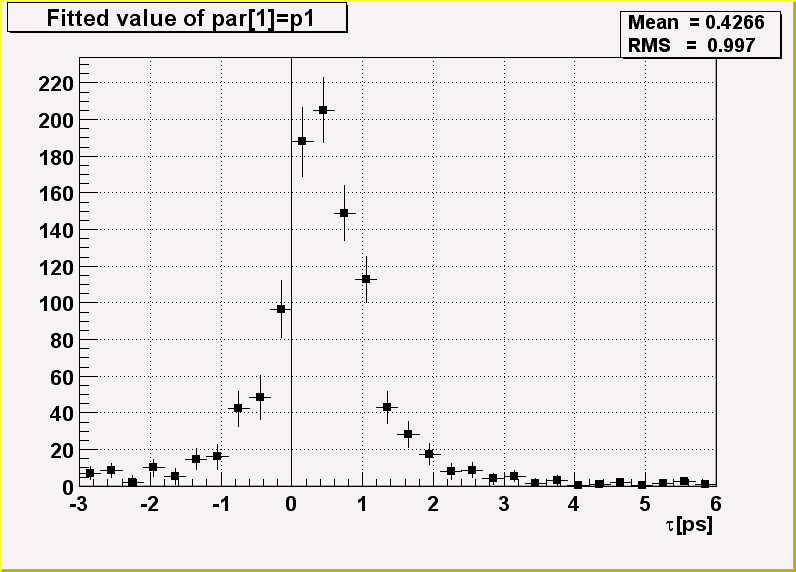# 23 Example Analysis

This chapter is an example of a typical physics analysis. Large data files are chained together and analyzed using the `TSelector` class.

## 23.1 Explanation

This script uses four large data sets from the H1 collaboration at DESY Hamburg. One can access these data sets (277 Mbytes) from the ROOT web site at: ftp://root.cern.ch/root/h1analysis/

The physics plots generated by this example cannot be produced using smaller data sets.

There are several ways to analyze data stored in a ROOT Tree

• Using `TTree::Draw`:

• This is very convenient and efficient for small tasks. A `TTree`::Draw call produces one histogram at the time. The histogram is automatically generated. The selection expression may be specified in the command line.

• Using the `TTreeViewer`:

• This is a graphical interface to `TTree::Draw` with the same functionality.

• Using the code generated by `TTree::MakeClass`:

• In this case, the user creates an instance of the analysis class. They have the control over the event loop and can generate an unlimited number of histograms.

• Using the code generated by `TTree::MakeSelector`: Like for the code generated by `TTree::MakeClass`, the user can do complex analysis. However, they cannot control the event loop. The event loop is controlled by `TTree::Process` called by the user. This solution is illustrated by the code below. The advantage of this method is that it can be run in a parallel environment using PROOF (the Parallel Root Facility).

A chain of four files (originally converted from PAW ntuples) is used to illustrate the various ways to loop on ROOT data sets. Each contains a ROOT Tree named “`h42`”. The class definition in h1analysis.h has been generated automatically by the ROOT utility `TTree``::MakeSelector` using one of the files with:

``h42->MakeSelector("h1analysis");``

This produces two files: h1analysis.h and `h1analysis.C`. A skeleton of `h1analysis.C` file is made for you to customize. The h1analysis class is derived from the ROOT class `TSelector`. The following members functions of h1analyhsis (i.e. `TSelector`) are called by the `TTree::Process` method.

• `Begin`: This function is called every time a loop over the tree starts. This is a convenient place to create your histograms.

• `Notify():` This function is called at the first entry of a new tree in a chain.

• `ProcessCut`: This function is called at the beginning of each entry to return a flag true if the entry must be analyzed.

• `ProcessFill`: This function is called in the entry loop for all entries accepted by Select.

• `Terminate`: This function is called at the end of a loop on a `TTree`. This is a convenient place to draw and fit your histograms.

To use this program, try the following session.

First, turn the timer on to show the real and CPU time per command.

``root[] gROOT->Time();``

Step A: create a `TChain` with the four H1 data files. The chain can be created by executed this short script `h1chain.C` below. \$H1 is a system symbol pointing to the H1 data directory.

``````{
TChain chain("h42");
chain.Add("\$H1/dstarmb.root");
//21330730 bytes, 21920 events
chain.Add("\$H1/dstarp1a.root");
//71464503 bytes, 73243 events
chain.Add("\$H1/dstarp1b.root");
//83827959 bytes, 85597 events
chain.Add("\$H1/dstarp2.root");
//100675234 bytes, 103053 events
}``````

Run the above script from the command line:

``root[] .x h1chain.C``

Step B: Now we have a directory containing the four data files. Since a `TChain` is a descendent of `TTree` we can call `TChain::Process` to loop on all events in the chain. The parameter to the `TChain::Process` method is the name of the file containing the created `TSelector` class (`h1analysis.C`).

``root[] chain.Process("h1analysis.C")``

Step C: Same as step B, but in addition fill the event list with selected entries. The event list is saved to a file “`elist.root`” by the `TSelector::Terminate` method. To see the list of selected events, you can do `elist->Print("all")`. The selection function has selected 7525 events out of the 283813 events in the chain of files. (2.65 per cent)

``root[] chain.Process("h1analysis.C","fillList")``

Step D: Process only entries in the event list. The event list is read from the file in `elist.root` generated by step C.

``root[] chain.Process("h1analysis.C","useList")``

Step E: The above steps have been executed with the interpreter. You can repeat the steps B, C, and D using ACLiC by replacing “`h1analysis.C`” by “`h1analysis.C+`” or “`h1analysis.C++`”.

Step F: If you want to see the differences between the interpreter speed and ACLiC speed start a new session, create the chain as in step 1, then execute

``root[] chain.Process("h1analysis.C+","useList")``

The commands executed with the four different methods B, C, D and E produce two canvases shown below:## 23.2 Script

This is the `h1analsysis.C` file that was generated by `TTree::MakeSelector` and then modified to perform the analysis.

``````#include "h1analysis.h"
#include "TH2.h"
#include "TF1.h"
#include "TStyle.h"
#include "TCanvas.h"
#include "TLine.h"
#include "TEventList.h"

const Double_t dxbin = (0.17-0.13)/40;   // Bin-width
const Double_t sigma = 0.0012;
TEventList *elist = 0;
Bool_t useList, fillList;
TH1F *hdmd;
TH2F *h2;

//_________________________________________________________
Double_t fdm5(Double_t *xx, Double_t *par)
{
Double_t x = xx;
if (x <= 0.13957) return 0;
Double_t xp3 = (x-par)*(x-par);
Double_t res = dxbin*(par*TMath::Power(x-0.13957,par)
+ par/2.5066/par*TMath::Exp(-xp3/2/par/par));
return res;
}

//_________________________________________________________
Double_t fdm2(Double_t *xx, Double_t *par)
{
Double_t x = xx;
if (x <= 0.13957) return 0;
Double_t xp3 = (x-0.1454)*(x-0.1454);
Double_t res = dxbin*(par*TMath::Power(x-0.13957,0.25)
+ par/2.5066/sigma*TMath::Exp(-xp3/2/sigma/sigma));
return res;
}

//_________________________________________________________
void h1analysis::Begin(TTree *tree)
{
// function called before starting the event loop
//  -it performs some cleanup
//  -it creates histograms
//  -it sets some initialization for the event list

//initialize the Tree branch addresses
Init(tree);

//print the option specified in the Process function
TString option = GetOption();
printf("Starting h1analysis with process option: %sn",option.Data());

//Some cleanup in case this function had already been executed
//Delete any previously generated histograms or functions
gDirectory->Delete("hdmd");
gDirectory->Delete("h2*");
delete gROOT->GetFunction("f5");
delete gROOT->GetFunction("f2");

//create histograms
hdmd = new TH1F("hdmd","dm_d",40,0.13,0.17);
h2   = new TH2F("h2","ptD0 vs dm_d",30,0.135,0.165,30,-3,6);

//process cases with event list
fillList = kFALSE;
useList  = kFALSE;
fChain->SetEventList(0);
delete gDirectory->GetList()->FindObject("elist");

// case when one creates/fills the event list
if (option.Contains("fillList")) {
fillList = kTRUE;
elist = new TEventList("elist","selection from Cut",5000);
}
// case when one uses the event list generated in a previous call
if (option.Contains("useList")) {
useList  = kTRUE;
TFile f("elist.root");
elist = (TEventList*)f.Get("elist");
if (elist) elist->SetDirectory(0);
//otherwise the file destructor will delete elist
fChain->SetEventList(elist);
}
}
//_________________________________________________________
Bool_t h1analysis::ProcessCut(Int_t entry)
{ // Selection function to select D* and D0.

//in case one event list is given in input,
//the selection has already been done.
if (useList) return kTRUE;
// Read only the necessary branches to select entries.
// return as soon as a bad entry is detected
b_md0_d->GetEntry(entry);
if (TMath::Abs(md0_d-1.8646) >= 0.04) return kFALSE;
b_ptds_d->GetEntry(entry);
if (ptds_d <= 2.5) return kFALSE;
b_etads_d->GetEntry(entry);
if (TMath::Abs(etads_d) >= 1.5) return kFALSE;
b_ik->GetEntry(entry);  ik--;
//original ik used f77 convention starting at 1
b_ipi->GetEntry(entry);
ipi--;
b_ntracks->GetEntry(entry);
b_nhitrp->GetEntry(entry);
if (nhitrp[ik]*nhitrp[ipi] <= 1) return kFALSE;
b_rend->GetEntry(entry);
b_rstart->GetEntry(entry);
if (rend[ik]-rstart[ik] <= 22)   return kFALSE;
if (rend[ipi]-rstart[ipi] <= 22) return kFALSE;
b_nlhk->GetEntry(entry);
if (nlhk[ik] <= 0.1)    return kFALSE;
b_nlhpi->GetEntry(entry);
if (nlhpi[ipi] <= 0.1)  return kFALSE;
b_ipis->GetEntry(entry);
ipis--;
if (nlhpi[ipis] <= 0.1) return kFALSE;
b_njets->GetEntry(entry);
if (njets < 1)          return kFALSE;

// if option fillList, fill the event list
if (fillList) elist->Enter(fChain->GetChainEntryNumber(entry));

return kTRUE;
}

//_________________________________________________________
void h1analysis::ProcessFill(Int_t entry)
{ // Function called for selected entries only

// read branches not processed in ProcessCut
b_dm_d->GetEntry(entry);
//read branch holding dm_d
b_rpd0_t->GetEntry(entry);
//read branch holding rpd0_t
b_ptd0_d->GetEntry(entry);
//read branch holding ptd0_d           //continued...
//fill some histograms
hdmd->Fill(dm_d);
h2->Fill(dm_d,rpd0_t/0.029979*1.8646/ptd0_d);
}

//_________________________________________________________
void h1analysis::Terminate()
{ // Function called at the end of the event loop

//create the canvas for the h1analysis fit
gStyle->SetOptFit();
TCanvas *c1 = new TCanvas("c1","h1analysis analysis",10,10,800,600);
c1->SetBottomMargin(0.15);
hdmd->GetXaxis()->SetTitle("m_{K#pi#pi}-m_{K#pi}[GeV/c^{2}]");
hdmd->GetXaxis()->SetTitleOffset(1.4);

//fit histogram hdmd with function f5 using
//the loglikelihood option
TF1 *f5 = new TF1("f5",fdm5,0.139,0.17,5);
f5->SetParameters(1000000,.25,2000,.1454,.001);
hdmd->Fit("f5","lr");

//create the canvas for tau d0
gStyle->SetOptFit(0);
gStyle->SetOptStat(1100);
TCanvas *c2 = new TCanvas("c2","tauD0",100,100,800,600);
c2->SetGrid();
c2->SetBottomMargin(0.15);

// Project slices of 2-d histogram h2 along X ,
// then fit each slice with function f2 and make a
// histogram for each fit parameter.
// Note that the generated histograms are added
// to the list of objects in the current directory.

TF1 *f2 = new TF1("f2",fdm2,0.139,0.17,2);
f2->SetParameters(10000,10);
h2->FitSlicesX(f2,0,0,1,"qln");
TH1D *h2_1 = (TH1D*)gDirectory->Get("h2_1");
h2_1->GetXaxis()->SetTitle("#tau[ps]");
h2_1->SetMarkerStyle(21);
h2_1->Draw();
c2->Update();
TLine *line = new TLine(0,0,0,c2->GetUymax());
line->Draw();

// save the event list to a Root file if one was produced
if (fillList) {
TFile efile("elist.root","recreate");
elist->Write();
}
}``````